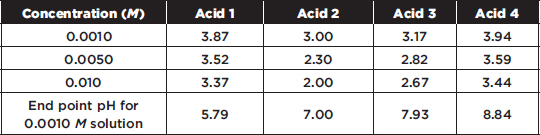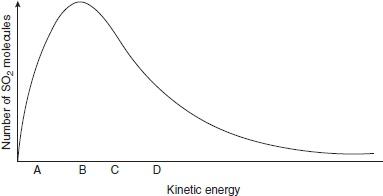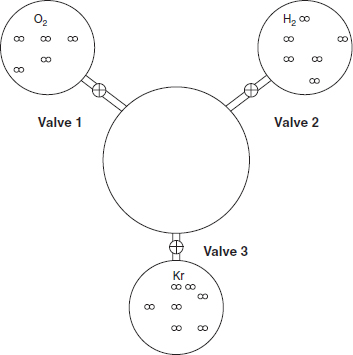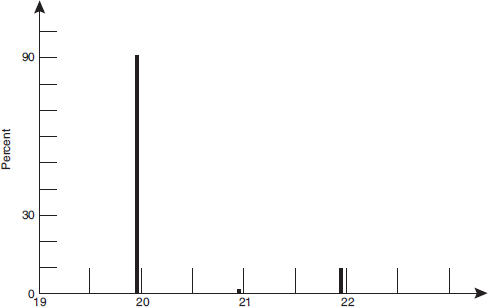# AP Chemistry Practice Test 27

### Test Information12 questions18 minutes

1. Sulfurous acid is a weak acid, while sulfuric acid is a much stronger acid because

2. You cannot prepare a buffer by

3.

 Photoelectron Spectroscopy Data Table Binding Energy (MJ) Relative Number of Electrons 0.58 1 1.09 2 7.19 6 12.1 2 151 2

The photoelectron data table for aluminum is shown above. Which of the following statements is not true about these data?

`Questions 4-7 refer to the following information.Four different acid solutions are prepared, and their pH values are determined and tabulated below.`

4. Using the data in the table above, which is the weakest acid?

5. Which of the four acids is best described as a strong acid?

6. Which of the following operations will produce an effective buffer solution?

7. Each of the acids were titrated with NaOH to the end point. The end point pH values are shown on the last line of the table. The end point pH values

8. An experiment was performed to determine the moles of hydrogen gas formed (collected over water) when an acid reacts with magnesium metal. To do this, a piece of dry magnesium was weighed. Then 50 mL of hydrogen was collected. Next the Mg was dried to remove about 0.1 mL of water and weighed again to see how much Mg had reacted. The volume of hydrogen was measured and converted into moles of hydrogen. Which mistake will give the largest error in the result?

9.The graph above shows the distribution of kinetic energies of a system containing a large number of SO2 molecules at 300 K. Which letter shows the average kinetic energy of this system?

10. A 25 g sample of a liquid was heated to 95 °C and then quickly transferred to an insulated container holding 100 g of water at 26 °C. The temperature of the mixture rose to reach a final temperature of 37 °C. Which of the following can be concluded?

11. Three 1-liter flasks are connected to a 3-liter flask by valves. The 3-liter flask is evacuated to start and the entire system is at 585 K. The first flask contains oxygen, the second hydrogen, and the third nitrogen. The pressure of hydrogen is 1.65 atm. The amounts of gas molecules are proportional to their representations in the flasks. If valve 2 is opened first and then the rest of the valves are opened, what will the pressure be after the first valve is opened and after they all are opened? Assume the connections have negligible volume.Valve 2 Opened   All Valves Opened

12.A mass spectrum of a naturally occurring sample of an element is shown above. What is the element?